Warning

This documents an unmaintained version of NetworkX. Please upgrade to a maintained version and see the current NetworkX documentation.

# Bipartite¶

This module provides functions and operations for bipartite graphs. Bipartite graphs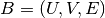have two node sets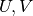and edges in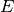that only connect nodes from opposite sets. It is common in the literature to use an spatial analogy referring to the two node sets as top and bottom nodes.

The bipartite algorithms are not imported into the networkx namespace at the top level so the easiest way to use them is with:

>>> import networkx as nx
>>> from networkx.algorithms import bipartite


NetworkX does not have a custom bipartite graph class but the Graph() or DiGraph() classes can be used to represent bipartite graphs. However, you have to keep track of which set each node belongs to, and make sure that there is no edge between nodes of the same set. The convention used in NetworkX is to use a node attribute named “bipartite” with values 0 or 1 to identify the sets each node belongs to.

For example:

>>> B = nx.Graph()
>>> B.add_edges_from([(1,'a'), (1,'b'), (2,'b'), (2,'c'), (3,'c'), (4,'a')])


Many algorithms of the bipartite module of NetworkX require, as an argument, a container with all the nodes that belong to one set, in addition to the bipartite graph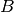. Ifis connected, you can find the node sets using a two-coloring algorithm:

>>> nx.is_connected(B)
True
>>> bottom_nodes, top_nodes = bipartite.sets(B)


list(top_nodes) [1, 2, 3, 4] list(bottom_nodes) [‘a’, ‘c’, ‘b’]

However, if the input graph is not connected, there are more than one possible colorations. Thus, the following result is correct:

>>> B.remove_edge(2,'c')
>>> nx.is_connected(B)
False
>>> bottom_nodes, top_nodes = bipartite.sets(B)


list(top_nodes) [1, 2, 4, ‘c’] list(bottom_nodes) [‘a’, 3, ‘b’]

Using the “bipartite” node attribute, you can easily get the two node sets:

>>> top_nodes = set(n for n,d in B.nodes(data=True) if d['bipartite']==0)
>>> bottom_nodes = set(B) - top_nodes


list(top_nodes) [1, 2, 3, 4] list(bottom_nodes) [‘a’, ‘c’, ‘b’]

So you can easily use the bipartite algorithms that require, as an argument, a container with all nodes that belong to one node set:

>>> print(round(bipartite.density(B, bottom_nodes),2))
0.42
>>> G = bipartite.projected_graph(B, top_nodes)
>>> G.edges()
[(1, 2), (1, 4)]


All bipartite graph generators in NetworkX build bipartite graphs with the “bipartite” node attribute. Thus, you can use the same approach:

>>> RB = bipartite.random_graph(5, 7, 0.2)
>>> RB_top = set(n for n,d in RB.nodes(data=True) if d['bipartite']==0)
>>> RB_bottom = set(RB) - RB_top
>>> list(RB_top)
[0, 1, 2, 3, 4]
>>> list(RB_bottom)
[5, 6, 7, 8, 9, 10, 11]


For other bipartite graph generators see the bipartite section of Graph generators.

## Basic functions¶

### Bipartite Graph Algorithms¶

 is_bipartite(G) Returns True if graph G is bipartite, False if not. is_bipartite_node_set(G, nodes) Returns True if nodes and G/nodes are a bipartition of G. sets(G) Returns bipartite node sets of graph G. color(G) Returns a two-coloring of the graph. density(B, nodes) Return density of bipartite graph B. degrees(B, nodes[, weight]) Return the degrees of the two node sets in the bipartite graph B.

## Matching¶

Provides functions for computing a maximum cardinality matching in a bipartite graph.

If you don’t care about the particular implementation of the maximum matching algorithm, simply use the maximum_matching(). If you do care, you can import one of the named maximum matching algorithms directly.

For example, to find a maximum matching in the complete bipartite graph with two vertices on the left and three vertices on the right:

>>> import networkx as nx
>>> G = nx.complete_bipartite_graph(2, 3)
>>> left, right = nx.bipartite.sets(G)
>>> list(left)
[0, 1]
>>> list(right)
[2, 3, 4]
>>> nx.bipartite.maximum_matching(G)
{0: 2, 1: 3, 2: 0, 3: 1}


The dictionary returned by maximum_matching() includes a mapping for vertices in both the left and right vertex sets.

 eppstein_matching(G) Returns the maximum cardinality matching of the bipartite graph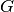. hopcroft_karp_matching(G) Returns the maximum cardinality matching of the bipartite graph. to_vertex_cover(G, matching) Returns the minimum vertex cover corresponding to the given maximum matching of the bipartite graph.

## Matrix¶

 biadjacency_matrix(G, row_order[, ...]) Return the biadjacency matrix of the bipartite graph G. from_biadjacency_matrix(A[, create_using, ...]) Creates a new bipartite graph from a biadjacency matrix given as a SciPy sparse matrix.

## Projections¶

One-mode (unipartite) projections of bipartite graphs.

 projected_graph(B, nodes[, multigraph]) Returns the projection of B onto one of its node sets. weighted_projected_graph(B, nodes[, ratio]) Returns a weighted projection of B onto one of its node sets. collaboration_weighted_projected_graph(B, nodes) Newman’s weighted projection of B onto one of its node sets. overlap_weighted_projected_graph(B, nodes[, ...]) Overlap weighted projection of B onto one of its node sets. generic_weighted_projected_graph(B, nodes[, ...]) Weighted projection of B with a user-specified weight function.

## Spectral¶

Spectral bipartivity measure.

 spectral_bipartivity(G[, nodes, weight]) Returns the spectral bipartivity.

## Clustering¶

 clustering(G[, nodes, mode]) Compute a bipartite clustering coefficient for nodes. average_clustering(G[, nodes, mode]) Compute the average bipartite clustering coefficient. latapy_clustering(G[, nodes, mode]) Compute a bipartite clustering coefficient for nodes. robins_alexander_clustering(G) Compute the bipartite clustering of G.

## Redundancy¶

Node redundancy for bipartite graphs.

 node_redundancy(G[, nodes]) Computes the node redundancy coefficients for the nodes in the bipartite graph G.

## Centrality¶

 closeness_centrality(G, nodes[, normalized]) Compute the closeness centrality for nodes in a bipartite network. degree_centrality(G, nodes) Compute the degree centrality for nodes in a bipartite network. betweenness_centrality(G, nodes) Compute betweenness centrality for nodes in a bipartite network.

## Generators¶

Generators and functions for bipartite graphs.

 complete_bipartite_graph(n1, n2[, create_using]) Return the complete bipartite graph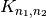. configuration_model(aseq, bseq[, ...]) Return a random bipartite graph from two given degree sequences. havel_hakimi_graph(aseq, bseq[, create_using]) Return a bipartite graph from two given degree sequences using a Havel-Hakimi style construction. reverse_havel_hakimi_graph(aseq, bseq[, ...]) Return a bipartite graph from two given degree sequences using a Havel-Hakimi style construction. alternating_havel_hakimi_graph(aseq, bseq[, ...]) Return a bipartite graph from two given degree sequences using an alternating Havel-Hakimi style construction. preferential_attachment_graph(aseq, p[, ...]) Create a bipartite graph with a preferential attachment model from a given single degree sequence. random_graph(n, m, p[, seed, directed]) Return a bipartite random graph. gnmk_random_graph(n, m, k[, seed, directed]) Return a random bipartite graph G_{n,m,k}.